Home | Introduction | Software | Examples | History | People | Bibliography | Applications | Theorems

# The Non-Hermitian Anderson Model

The non-Hermitian Anderson model was first proposed by Hatano and Nelson for the analysis of vortex pinning in type-II superconductors . This model has also been applied to the study of population dynamics .

The relevant "stochastic circulant" matrix is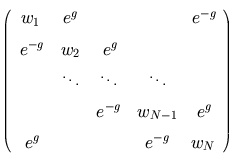,
where g is a constant and the diagonal entries are drawn from some fixed probability distribution. All unspecified entries are zero.

The circulant structure arises because the model describes the motion of a particle along a ring. The eigenvalues of this matrix (for appropriate probability distributions) form a "bubble with wings" in the complex plane. Feinberg and Zee [2,3] and Goldsheid and Khoruzhenko  have studied the location of the curves containing the eigenvalues, and the eigenvalue density on that curve. The eigenvalues for such an example are shown in Figure 1. In the infinite dimensional case, the eigenvalues of the corresponding Laurent operator have been studied by Davies .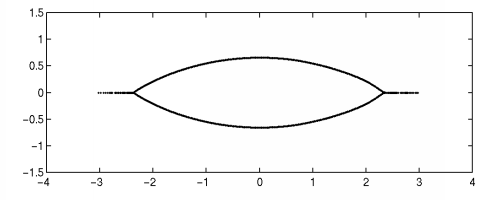Figure 1. Spectrum for one realization of the (circulant) non-Hermitian Anderson model of dimension N=1000 with g=0.4. The diagonal entries are uniformly distributed in [-1.5, 1.5].

The circulant non-Hermitian Anderson model typically exhibits a mild degree of non-normality, as can be seen in Figures 2 and 3 below. For small values of epsilon, the pseudospectra are located quite close to the "bubble with wings"; in particular, note that the pseudospectra have a hole in the middle.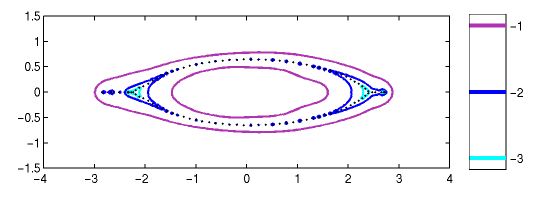Figure 2. Spectrum and epsilon-pseudospectra for a (circulant) non-Hermitian Anderson model of dimension N=100 with g=0.4. The diagonal entries are uniformly distributed in [-1.5, 1.5]. The values on the colorbar show log10(epsilon).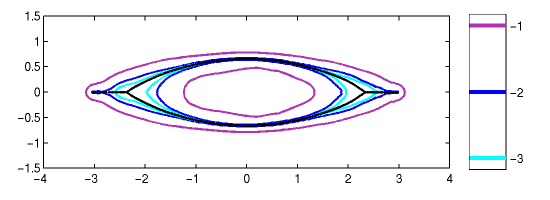Figure 3. Spectrum and epsilon-pseudospectra for a (circulant) non-Hermitian Anderson model of dimension N=1000 with g=0.4. The diagonal entries are uniformly distributed in [-1.5, 1.5]. The values on the colorbar show log10(epsilon).

One can imagine instances where the ring structure that makes the above model periodic is inappropriate. If we remove the corner entries induced by that periodicity,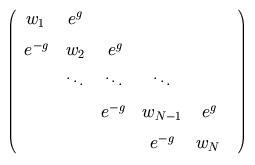(and if the diagonal entries are real), then all the eigenvalues must be real. Yet this matrix is highly non-normal and, for sufficiently large dimensions, the borders of the pseudospectra capture the "bubble with wings" structure observed for the matrix with corner entries. Pseudospectra for two such matrices are shown in Figures 3 and 4 below.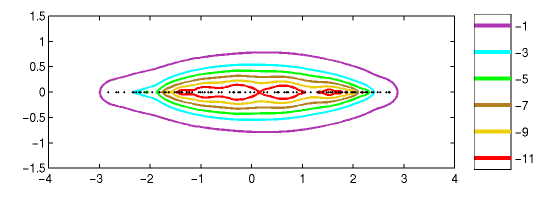Figure 4. Spectrum and epsilon-pseudospectra for a (non-circulant) non-Hermitian Anderson model of dimension N=100 with g=0.4. The diagonal entries are uniformly distributed in [-1.5, 1.5]. The values on the colorbar show log10(epsilon).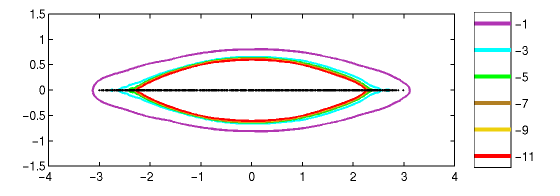Figure 5. Spectrum and epsilon-pseudospectra for the (non-circulant) non-Hermitian Anderson model of dimension N=1000 with g=0.4. The diagonal entries are uniformly distributed in [-1.5, 1.5]. The values on the colorbar show log10(epsilon).

# Bibliography

  E. B. Davies, Spectral properties of random non-self-adjoint matrices and operators, Proc. Roy. Soc. Lond. Ser. A, to appear. See math/0002159.  J. Feinberg and A. Zee, Non-Hermitean localization and de-localization, Phys. Rev. E 59 (1999), 6433-6443. See cond-mat/9706218.  J. Feinberg and A. Zee, Spectral curves of non-hermitian hamiltonians, Nuc. Phys. B 522 (1999), 599-623. See cond-mat/9710040.  I. Ya. Goldsheid and B. A. Khoruzhenko, Distribution of eigenvalues in non-Hermitian Anderson models, Phys. Rev. Lett. 80 (1998), 2897-2900. See cond-mat/9707230.  N. Hatano and D. R. Nelson, Localization transitions in non-Hermitian quantum mechanics, Phys. Rev. Lett. 77 (1996), 570-573. See cond-mat/9603165.  D. R. Nelson and N. M. Shnerb, Non-Hermitian localization and population biology, Phys. Rev. E 58 (1998), 1383-1403. See cond-mat/9708071.